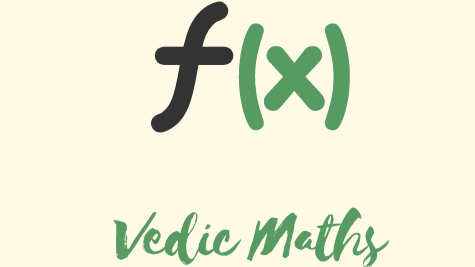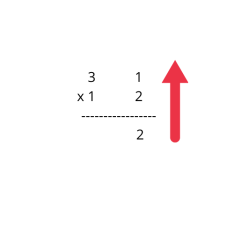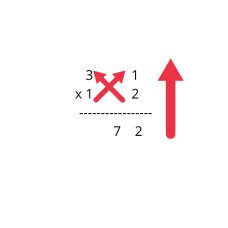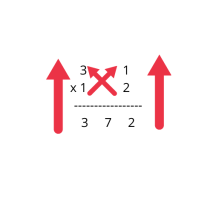# Vedic Maths :- multiplying two 2-digit numbers.

By ac05 | Vedic Maths | 5 May 2021

When you will know this awesome trick, then you will be able to perform the multiplication of two 2-digit numbers easily without using a calculator. Let's understand how to perform this by taking the example 31 x 12.

• Multiply the ones digit vertically• Multiplying in a cross pattern and adding the results.• Multiplying the tens digit vertically.This is how one can calculate the product of two 2-digit numbers easily.

Important Note :-

If while performing the second step, cross multiplication and addition, the results appears to be two-digit number take carry the 10's digit and add it to step 3. Example

in 34 * 34 the second step would result in 24 so 2 will be carried and added to step 3.

Try some problems on your own.

• 34*34
• 23*12
• 10*17ac05

A competitive Programmer exploring different domainsVedic Maths

Vedic mathematics consists of various techniques that makes calculations easy and fast. Well i will be posting some of the vedic maths tricks, made by ancient Indian mathematics. If you grasp those tricks you could easily do calculation in your mind. Now you won't need calculator every time to do addition or multiplication or squaring or even square rooting a number. At the end of each post I will be adding few questions that you must do with those tricks without using calculator. Have a nice day!Send a \$0.01 microtip in crypto to the author, and earn yourself as you read!

20% to author / 80% to me.
We pay the tips from our rewards pool.